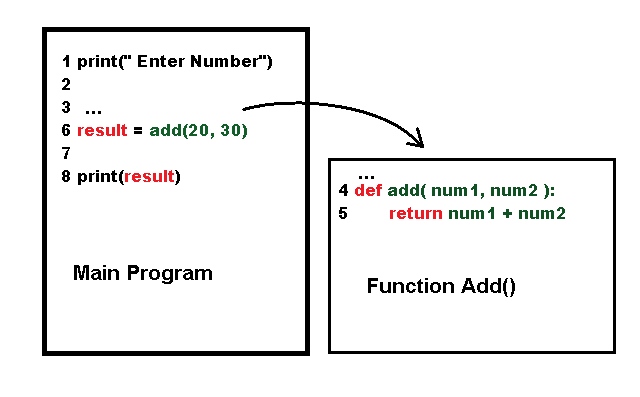# Python Functions

25 total views

So far we have seen many programs which ran sequentially, means executed the lines of codes in a linear manner. We have also seen blocks of code such as if-then-else and loops such as for-loop and while-loop. In this article , you learn about python functions.

Once thing is common to all of these codes, that is, re-usability. None of the above is reusable. You cannot run the same loop or if-block in two different places.

Contents

### Python Functions

Python functions are named block of code that you can reuse in multiple places in your program. Python uses different types of function, you can create new variables within the function, also functions may or may not return results. The function also take parameters.

### How Functions Work?

The main program executes and knows that a function definition exists. When the program need to call the function to do a specific task. It calls it and transfer the control over to the function. Note that in python function definition always comes before function call.The main program pass control to the function which return the control back to main program.

In the figure above, line 4 and 5 is not executed until line 6 do the function call. You can say that the function is a program by itself. The transfer of control to the function is the non-sequential nature of functional programming which is a different programming paradigm altogether.

### Types of Functions in Python

There are three types of functions in python programming. They are:

1. Built-in Functions
2. Import Class Methods
3. User-defined functions

In the next few sections, we will talk about the types of function in detail.

### Built-in Functions in Python

Several functions are built-in to the python and very useful. You have seen one such function – print(). All of these function may or may not take some arguments and return an output.

For example.

## The print() function will simply print the content to the console.
print(" This is a string")
## The abs() function is called the absolute function will return an
## absolute value of an integer.
s1 = abs(4)
print(s1)
# output 4
s2 = abs(-4)
print(s2)
# output 4

### Imported Module Methods

Methods are also type of function except that they are tied to an object and class. Python allows use to import modules which has a set of function that you can use for specific purpose.For example, the math module has several mathematical functions.

# program to find the square root
import math
num = 144
print(math.sqrt(num))

Output of the above program is square root of the number 144.

12.0

### User-Defined Function in Python

The main advantage of python is the ability to create your own function also called the user-defined function. The programmers can write their own functions.

Function Definition

Function definition is the code which defines what a function can do. It can calculate an expression, print something, or manipulate input data and return the result to the main program.

def multiply(n1, n2):
return n1 * n2

The function definition always starts with the keyword – def, followed by a function name – here it is multiply(). This part is called function header.

The function may or may not take parameters within its parenthesis (). In the example above, the function takes two parameters – n1 and n2.

The second part of the function is its body. The function do some evaluation or computation in the function body. After evaluation or performing a specific task, a function may or may not return a result. The keyword return indicate that the function is returning a value.

In the above example, the function is returning the value after multiplying n1 and n2.

Function Call

The function definition is tells the program what it does, however, to use a function the main program or a function must call another function. This is known as function call.

If the function has parameters, then user must provide some arguments to the function. An argument replaces the parameter in the function and it is evaluated within the function body.

Consider the following example.

# Function definition
def multiply(n1, n2):
return n1 * n2
# Function call
result = multiply(24, 10)
# Print output
print(he result is", result)

The output of the program is given below.

=== RESTART: C:/Python_projects/Functions/Function_Example.py ====
The result is 240
>>> 

In the next article, we will discuss different structures of functions in detail.

Bestseller

Python Programming: Using Problem Solving Approach

Python Programming is designed as a textbook to fulfil the requirements of the first-level course in Python programming. It is suited for undergraduate degree students of computer science engineering, information technology as well as computer applications. This book will enable students to apply the Python programming concepts in solving real-world problems. The book begins with an introduction to computers, problem solving approaches, programming languages, object oriented programming and Python programming. Separate chapters dealing with the important constructs of Python language such as control statements, functions, strings, files, data structures, classes and objects, inheritance, operator overloading and exceptions are provided in the book. Each chapter ends with objective-type questions, review questions, programming and debugging exercises to facilitate revision and practice of concepts learnt.